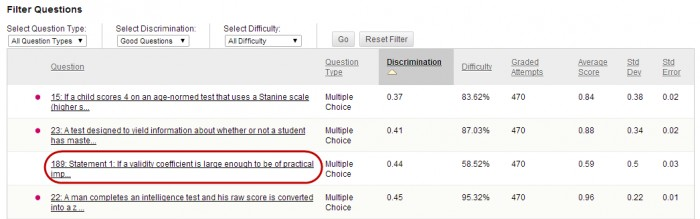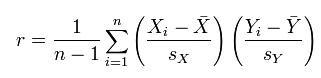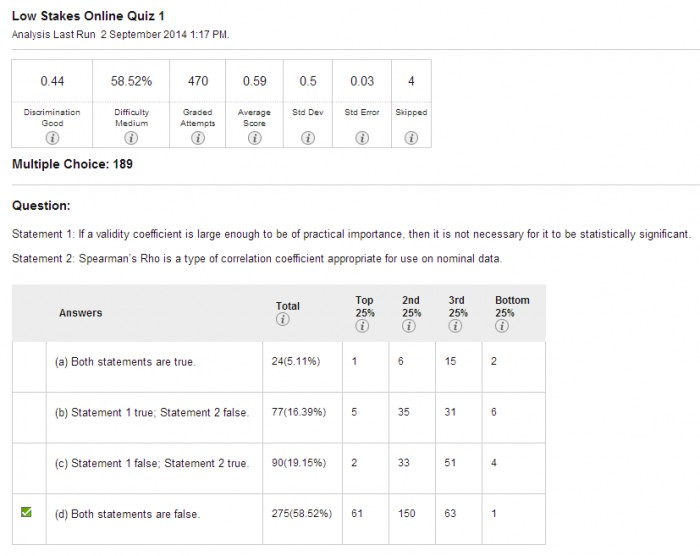The results of individual questions can be analysed when you run an Item Analysis (refer Run Item Analysis guide).

## Question Statistics Table

1. Click on the Show All button to show all the questions.
2. Click on the link of the required question.## Individual question

### Columns

• Discrimination: Indicates how well a question differentiates between students. A question is considered a good discriminator when students who answer the question correctly also do well on the rest of the test.
• Values can range from -1.0 to +1.0 Questions are flagged for review with a red dot if their discrimination value is less than 0.1. Discrimination values cannot be calculated when the question’ difficulty score is 100% or when all student receive the same score on a question.

Calculation: Discrimination values are calculated with the Pearson correlation coefficient, X represents the scores of each student on a question and Y represents the scores of each student on the test.• Difficulty: The percentage of students who answered the question correctly. Difficulty values can range from 1% to 100% with a high percentage indication that the question was easy.

Note: A high difficulty value does not assure a high level of discrimination.

• Grade Attempts: Number of questions attempts where marking is complete. i.e. Short answer, Essay and File response question types need to be marked by staff.
• Average Score: The score displayed is the average score reported for the test in the Grade Centre. Scores with a * included tests that have not been marked and the average may change after all tests have been marked.
• Standard Deviation: Measure of how far the scores deviate from the average score.
• If the scores are tightly grouped, with most of the values being close to the average, the standard deviation is small.
• If the data set is widely dispersed, with values far from the average the standard deviation is larger.
• Standard Error: An estimate of the amount of variability is a student’s score due to chance. The smaller the standard error of measurement, the more accurate the measurement provided by the test question.
• Skipped: The number of students who skipped this question.### Question statistics

The information shown in these sections varies depending on the question type.

Type of information provided

Question types

Number of students who selected each answer choice.

AND

Distribution of those answers among the class quartiles

• Multiple Choice
• True / False
• Either / Or
• Opinion Scale / Likert

Numberer of students who selected each answer choice.

• Matching Choice
• Ordering
• Fill in the Multiple Blanks

Number of students who got the question correct, incorrect or skipped it.

• Calculated Formula
• Calculated Numeric
• Fill in the Blank
• Quiz Bowl

Question test only

• Essay
• File Response